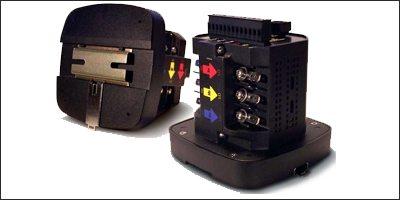# PRECISION DIGITAL AC POWER CONTROLLER

This is a circuit for a digital AC power controller that can set different firing times corresponding to different power angles. -- PRATAP CHANDRA SAHU

2456SCRs and Triacs are extensively used in modern electronic power controllers—in which power is controlled by means of phaseangle variation of the conduction period. Controlling the phase angle can be made simple and easy if we set different firing times corresponding to different firing angles. The design given here is a synchronised programmable timer which achieves this objective.

The following equation for a sinewave shows how firing time and the phase angle are related to each other:

`θ = 2πft or θ∝t`

Here, θ is the angle described by a sinewave in time t (seconds), while f is the frequency of sinewave in Hz. Time period T (in seconds) of a sinewave is equal to the reciprocal of its plies a narrow positive pulse which resets the timer at every zero crossing.

A 32.768kHz crystal is used to get stable output of nearly 1 kHz (1,024Hz) frequency after five stages of binary division by an oscillator-cum-divider IC CD4060. The 32.768kHz crystal is used because it can be found in unused quartz clocks and is readily available in the market. But use of a 1kHz crystal using a quad-NAND IC frequency, i.e. T = 1/f.

The above equation indicates that if one divides the angle described during one complete cycle of the sinewave (2π = 360o) into equal parts, then time period T of the wave will be divided into identical equal parts. Thus, it becomes fairly easy to set the different programmable timings synchronised with the AC mains sinewave at zero crossing. The main advantage of such an arrangement, as already mentioned earlier, is that only the firing time has to be programmed to set different firing angles. It is to be noted that the more precise the timer, the more precise will be the power being controlled.

In this circuit, the time period of mains waveform is divided into 20 equal parts. So, there is a time interval of 1 ms between two consecutive steps. The sampling voltage is unfiltered full-wave and is obtained from the diode bridge at the output of the power transformer. The timer is reset at every zero crossing of full wave and is obtained from the diode bridge at CD4093 as clock generator, as shown in the output of the power transformer. The timer is reset at every zero crossing of full wave and Fig. 2, is better as it provides the exact time interval required. In that case, CD4060 oscillator/divider is not required.

The CD4017B counter-cum-decoder IC then divides this 1kHz signal into ten equal intervals, which are programmed via the single-pole, 10-way rotary switch. Once the delayed output reaches the desired time interval, the corresponding output of CD4017 inhibits the counter CD4017 (via set again instantly for the next delay time. This arrangement helps the timer to be set for every half of mains wave—when the positive half of the mains waveform starts building up, the timer is set for that half and as it begins to cross zero, it gets reset and set again for negative half, when the negative half begins to build up. The process is repeated. Here, instead of using two zero crossing detectors—one for each half of mains wave—a single detector is used to perform both the functions. This is possible because the sampling wave for negative half is inverted by the rectifier diode bridge.

The 18V AC from power transformer is fed to the four diodes in bridge configuration, followed by the filter capacitor which is again followed by a threeterminal voltage regulator IC LM7812.The voltage so obtained drives the circuit. The unfiltered voltage is isolated from the filter capacitorby a diode and is fed to zener diode D8, which acts as a clipper to clip voltage above 6 volts.

This voltage is fed to the base of transistor T1, which is wired as zero crossing detector. When base voltage reaches the threshold, it conducts. It thus sup pole of rotary switch and diode D6) and fires the Triac. Transistor T2 here acts as a driver transistor. The reset pin of 4017 is connected to zero crossing detector output to reset it at every zero crossing. (The load-current waveforms for a few positions of the rotary switch, as observed at EFY Lab, are shown in Fig. 3.)

The circuit can be used as power controller in lighting equipment, hot-air oven, universal single-phase AC motor, heater, etc.

1.N.S.RAO